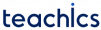# Pattern matching algorithms

Pattern matching finds whether or not a given string pattern appears in a string text. Commonly used pattern matching algorithms are Naive Algorithm for pattern matching and pattern matching algorithm using finite automata.

### Naive Algorithm for pattern matching

PAT and TEXT are two strings with length R and S respectively. This algorithm finds INDEX(P)

1. SET K=1 and MAX=S-R+1.
2. Repeat Step 3 to 5 while K<=MAX:
3.   Repeat for L=1 to R:
If TEXT[K+L-1] ≠ PAT[L], then: Go to Step 5.
4.   SET INDEX=K, and EXIT.
5.   K=K+1.
6. SET INDEX=0.
7. Exit.

The complexity of the pattern can be measured by the number of comparisons between characters in pattern PAT and characters of string TEXT. Let Nk denote the number of comparisons takes place inside the inner loop when PAT is compared with substring Wk.Here L is the position of where first PAT appears, or L=MAX if PAT doesn’t appear in TEXT.

The data size for the algorithm, when length of TEXT is s and length of PAT is r, is,When every character of PAT expect the last matches every SUBSTRING Wkwe know that, s=n-r.the maximum value of C(n) occurs when r=(n+1)/4. Substituting the value of r in the formula,### Pattern matching algorithm using finite automata

The second algorithm uses a table which is derived from pattern PAT independent of TEXT.

The pattern matching table F(Q,TEXT) of pattern PAT is in memory. The input is an N character string TEXT=T1, T2,…, Tn. This algorithm finds INDEX(PAT) in TEXT.

1. SET K=1 and MAX=S-R+1.
2. Repeat Step 3 to 5 while K<=MAX:
3.   Repeat for L=1 to R:
If TEXT[K+L-1] ≠ PAT[L], then: Go to Step 5.
4.   SET INDEX=K, and EXIT.
5.   K=K+1.
6. SET INDEX=0.
7. Exit

The figure contains the pattern matching table and pattern matching graph used in the algorithm for matching the pattern PAT=aaba.

The table is obtained as follows. First of all, we let Qi denote the initial substring of PAT of length i; henceThe rows are labelled by these initial substrings of P. The columns are labelled a,b and x, where x represents any character that doesn’t appear in the pattern PAT. Let f(Qi,t) be the function that denotes the entry in the table in row Qi and column t. For example, f(Q2,a)=Q2, f(Q3,x)=Q0…etc
This table can be pictured by a labelled directed graph as in the figure. Each node in the graph represents each initial substring Qi of PAT. These are called states of the system. There is an arrow in the graph corresponding to each entry in the table. For example, f(Q2,b)=Q3, so there is an arrow labelled b from Q2 to Q3. We have omitted all arrows labelled x that must lead to Q0.

Beginning with the initial state Q0 and using the text TEXT, we will obtain a sequence of states S1, S2, S3,… as follows. There are two possibilities here,

1. Some state Sk=PAT
2. No state S1, S2,…, SN+1 is equal to PAT.

In the first case, P does appear in T and its index is K-LENGTH(PAT). In the second case, P doesn’t appear in T.
The running time of this algorithm is proportional to the number of times the loop in the second step is executed. The worst-case occurs when the loop is executed LENGTH(TEXT) times. Then we can say that the complexity of this pattern matching algorithm is equal to O(n).

Subscribe
Notify of